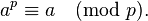Hackerrank Nov Challenge – Power of Large Numbers

PROBLEM STATEMENT

In a nutshell, we have a^b mod 10^9 + 7 ( ^ denotes power )

Range of a & b: 1 <= a,b <= 10^100000

PRE – REQUISITES

FERMAT’S THEOREM:

The theorem stats that, if is a prime number, then for any arbitrary integerMODULAR EXPONENTIATION:

Consider example of finding 5^13. 13 in binary format is 1101.

5^13 can split-ted into 5^8  *  5^*5^1

Pseudo code:

int findPower(base,power)

{

if( power % 2 == 1 )

res = res * base

power = power / 2

base = base * base

}

MODULAR ARITHMETIC

We need to aware of basic arithmetic properties like (a*b) mod m = ( (a mod m) * ( b mod m ) ) mod m

SOLUTION:

First step is we need to reduce both base and power so that they fit in the 32 – integer range.

Base value greater than 1o^9 + 7 can reduced by taking Modulo 1o^9 + 7. Based on Fermat’s theorem, Power value can be reduced by taking Modulo 10^9+6.

After reducing them to integers, find base^power using Modular Exponentation

CODE:

#include <cmath>
#include <cstdio>
#include <vector>
#include <string>
#include <iostream>
#include <algorithm>
using namespace std;

#define MOD (1000000007)

int findPow(int base,int power)
{
int ans = 1,basePower = base;

while( power > 0)
{
if( (power&1) == 1 )
ans = ( (long long int)ans * (long long int)basePower ) % (MOD);

basePower = ((long long int)basePower * (long long int)basePower) % (MOD);
power = power>>1;
}

return ans;
}

int findRem(string a,int num)
{
int sz = a.size(),i=0,rem=0,temp=0;
while( i < sz )
{
rem = ( ( (long long int)rem * 10LL ) % (num) + (a[i]-'0') ) % (num);
i++;
}
return rem;
}

int main() {
std::ios_base::sync_with_stdio(false);
int t;
cin>>t;
string a,b;
while(t--)
{
cin>>a>>b;

int x = findRem(a,(MOD));
int y = findRem(b,(MOD-1));
cout<<findPow(x,y)<<endl;
}
return 0;
}